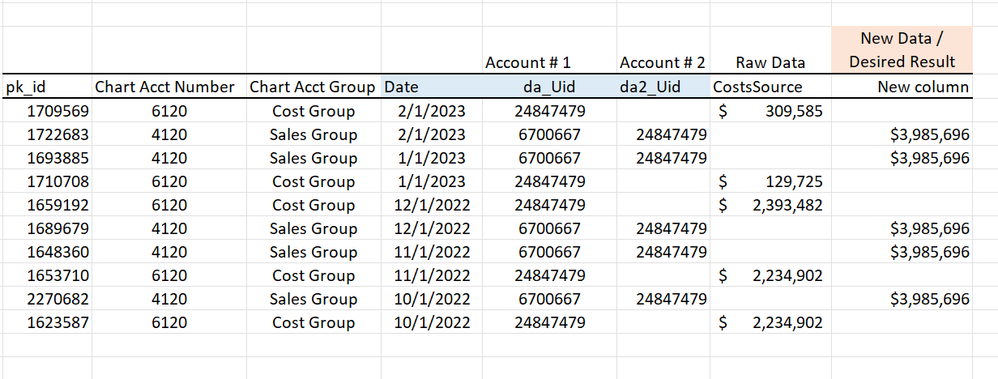cancel
Showing results for
Did you mean:Frequent Visitor

## Calculate Logic - Gross Margin Logic

Hi,

Can someone help me with this Gross Margin Logic. I can's seem to figure it out.

I want to get the Gross Margins of Sales account 4130, but the cost is on a different row. There is a Offset Account Number 2. Can I get a logic that calls Calculate or a Sum of Cost Column in the row above with the offset Acct Number.

GM \$ =  Calculate([Cost \$],

if Acct_Number 2 = Account Number with my data Below.

Thanks for any Help!4 REPLIES 4Frequent Visitor

I am so close to getting the correct result I want.

Can I just put a filter on this to get the correct Associated Date @ Account #2 with the Cost Source in this Calculated Column?

Here is my Calculated Column. It has the Cost Source in the right rows, but just I need the correct Cost Source Data associated with that Account #2 and Date.

Thanks for the Help!

New column =
maxx(filter(MonthlyFinancials,
MonthlyFinancials[dmf_AccountUid] = earlier(MonthlyFinancials[da_offset_id]) ),
MonthlyFinancials[CostsSource])Super User

@TBoat , You need to have one account table with a single name, may max of name.

Join this table with both account 1 and account2, If they are in the same table one join will inactive

Then you can create measure like , Assume account 1 is active join

calculate(Sum(Table[Cost]), isblank([Account 2]))

calculate(Sum(Table[Sales]), userelationship(Account[Account[, Table[Account2]), not(isblank([Account 2])) )

Else you have use earlier to move data across rows

Power BI DAX- Earlier, I should have known Earlier: https://youtu.be/CVW6YwvHHi8

New column = Sumx(filter(Table, Table[Account] = earlier(Table[Account2]) ), Table[Cost])

Then you have ignore few  rows

!! Microsoft Fabric !!
Microsoft Power BI Learning Resources, 2023 !!
Learn Power BI - Full Course with Dec-2022, with Window, Index, Offset, 100+ Topics !!
Did I answer your question? Mark my post as a solution! Appreciate your Kudos !! Proud to be a Super User! !!Frequent Visitor

Thanks for getting back to me @amitchandak I tried these solutions.

What is would the measure be if I wanted to get the Cost \$ amount on the associated Sales Line?

• I created a seperate Dim Table with relationship to Account to Fact Table Account , then Inactive Relationship from Dim Table Account  to Fact Table Account 2.

Measure

Cost Offset \$ CY = calculate(sum(Fact Table[SalesSource]),

USERELATIONSHIP(Dim Table[Account],Fact Table[Account 2]),
not(isblank(Fact Table[Account 2])))

This returns the Sales Number still. I want the Cost number, how do I get that in place of this? It is on a different Row. Shouldnt I sum costs? But then it doesnt have the reference of the Sales number. Not sure what I am missing.

Thanks!Frequent Visitor

Still not sure how to move the data row down with Userelationship or Treatas. Or if this is possible.